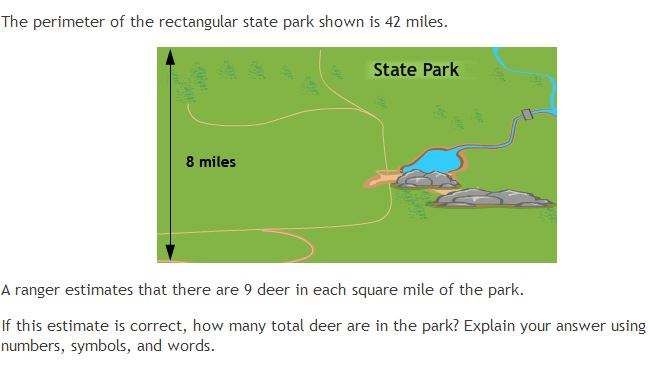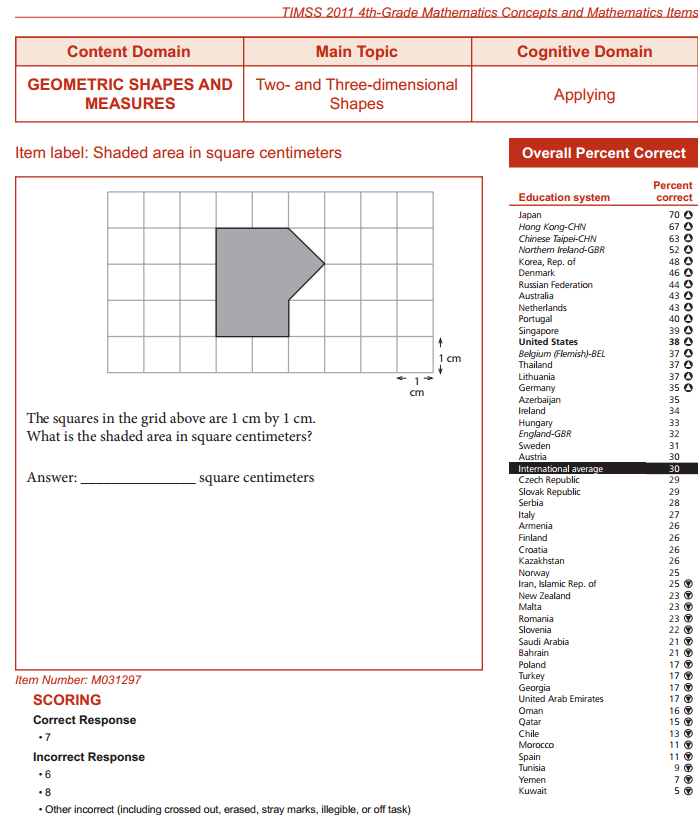### What Should a 4th Grader Know in Math?

Almost forty years have passed since I took 4th grade math. I remember being asked to calculate how long a light year is in miles but that was already at the beginning of 5th grade. A light year is the distance light has traveled in one year so it requires knowledge of how fast light moves and how much time there is in one year. In this case, it only requires the relationship between speed, distance and time. There is no geometry required. Fourth grade math under the Common Core not only includes measurements and conversions between measurements, but also two-dimensional shapes. Looking at a prototype of a question developed by the Partnership for Assessment of Readiness for College and Careers (PARCC), the difference between Common Core's 4th grade math and the one I had forty years ago becomes much more obvious:Above copied from The Mathematics Common Core Toolbox
The above question is indeed quite different from the tests I had when I was in 4th grade. In fact, it looks different from questions in the international standardized TIMSS exam for grade 4. Here is a sample question from TIMSS:Above copied from TIMSS 2011
With the above TIMSS question, all the student needs to do is count the number of squares that have been shaded. There are six completely shaded squares and there are two squares that are each half-shaded. Thus, the correct answer is seven square centimeters. This is a lot more straightforward and yet, only 38 percent of US students answered this question correctly.

Improving math education obviously involves much more than just writing new test questions. First, one must take a look at what current assessments are telling us. Grade 4 students are currently finding the above TIMSS question already challenging. This question only requires finding how much area is shaded. Among all the education systems in the world that participated in TIMSS 2011, there were only four that scored 50% or above on this question. The international average is suggesting that more than two-thirds of grade 4 students are currently unable. Thus, it is not surprising that on the Education Week blog where the above PARCC question is also presented, there is one comment posted:
"Unreasonable is the only way I can describe these questions."
By the way, to answer the question of how many deer are in the park, one must know the following:

• the perimeter of a rectangle is the sum of its four sides
• a rectangle is defined by two dimensions: length (L) and width (W)
• the perimeter of a rectangle is therefore equal to 2L + 2W
• from the given length and perimeter: 42 = 2(8) + 2W
• the width is therefore 13 miles
• the area of a rectangle is the product of its length and width, so in this case, the area is 8 times 13 = 104 square miles
• with 9 deer for every square mile, there are 104 times 9 deer in this park = 936 deer.